# 《NLP情感分析》（二）——Baseline

## 1.1 简介

```MDb数据集来源： @InProceedings{maas-EtAl:2011:ACL-HLT2011, author = {Maas, Andrew L. and Daly, Raymond E. and Pham, Peter T. and Huang, Dan and Ng, Andrew Y. and Potts, Christopher}, title = {Learning Word Vectors for Sentiment Analysis}, booktitle = {Proceedings of the 49th Annual Meeting of the Association for Computational Linguistics: Human Language Technologies}, month = {June}, year = {2011}, address = {Portland, Oregon, USA}, publisher = {Association for Computational Linguistics}, pages = {142--150}, url = {http://www.aclweb.org/anthology/P11-1015} }```

## 1.2 数据预处理

TorchText 的主要概念之一是`Field`，这些定义了应如何处理数据。 我们的数据集为带标签数据集，即数据由评论的原始字符串和情感组成，“pos”表示积极情绪，“neg”表示消极情绪。

`Field` 的参数指定应该如何处理数据。

`LABEL``LabelField` 定义，它是专门用于处理标签的 `Field` 类的特殊子集。稍后我们将解释 `dtype` 参数。

```Python import torch from torchtext.legacy import data

SEED = 1234

# 设置种子

torch.manual_seed(SEED)

# 将这个 flag 置为True的话，每次返回的卷积算法将是确定的，即默认算法。如果配合上设置 Torch 的随机种子为固定值的话，应该可以保证每次运行网络的时候相同输入的输出是固定的

torch.backends.cudnn.deterministic = True

# 读取数据和标签

TEXT = data.Field(tokenize = 'spacy', tokenizer_language = 'en_core_web_sm') LABEL = data.LabelField(dtype = torch.float) ````TorchText` 的另一个方便的功能是它支持自然语言处理 (NLP) 中使用的常见数据集。

```Python from torchtext.legacy import datasets

train_data, test_data = datasets.IMDB.splits(TEXT, LABEL) ```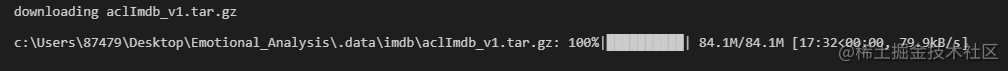查看我们的训练集和测试集大小：

```Python print(f'Number of training examples: {len(train_data)}') print(f'Number of testing examples: {len(test_data)}')```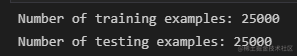```python print(vars(train_data.examples))```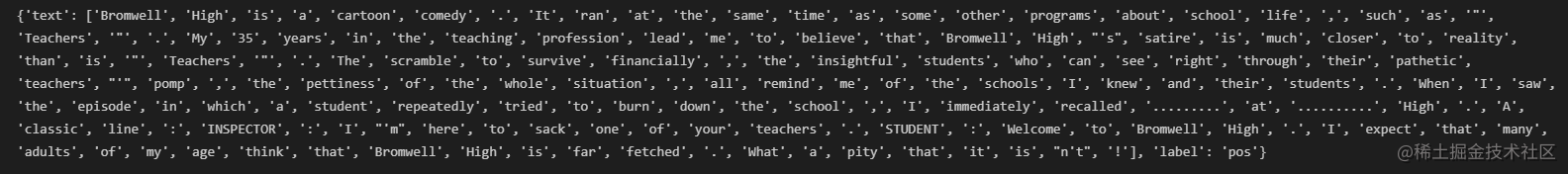IMDb 数据集划分了训练集和测试集，这里我们还需要创建一个验证集。 可以使用 `.split()` 方法来做。

```Python import random

train_data, valid_data = train_data.split(split_ratio=0.8 , random_state = random.seed(SEED)) ``` 现在我们看一下训练集，验证集和测试集分别有多少数据

```Python print(f'Number of training examples: {len(train_data)}') print(f'Number of validation examples: {len(valid_data)}') print(f'Number of testing examples: {len(test_data)}')```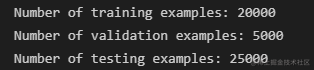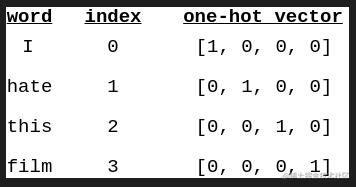```Python MAX_VOCAB_SIZE = 25000

TEXT.build_vocab(train_data, max_size = MAX_VOCAB_SIZE) LABEL.build_vocab(train_data) ```

```Python print(f"Unique tokens in TEXT vocabulary: {len(TEXT.vocab)}") print(f"Unique tokens in LABEL vocabulary: {len(LABEL.vocab)}")```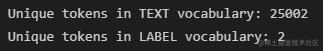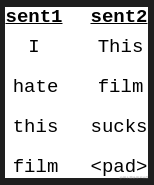```Python print(TEXT.vocab.freqs.most_common(20))```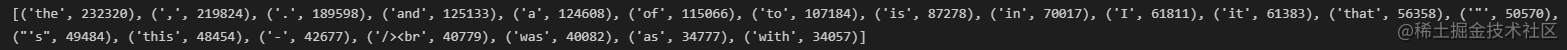```Python print(TEXT.vocab.itos[:10])```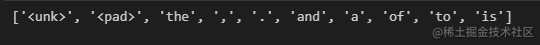```Python BATCH_SIZE = 64

device = torch.device('cuda' if torch.cuda.is_available() else 'cpu')

train_iterator, valid_iterator, test_iterator = data.BucketIterator.splits( (train_data, valid_data, test_data), batch_size = BATCH_SIZE, device = device) ```

## 1.3 构建模型

`__init__` 我们定义的模型的层数. 三层模型分别是嵌入层，RNN层，最后还有全连接层. 所有层的参数初始化都是随机的，除非对某些参数进行特别设置。 embedding层将稀疏的one-hot转换为密集嵌入到空间的向量.embedding 层是一个简单的单层的全连接层. 这样也减少了输入到RNN的维数，减少了数据的运算的计算量,这里有一种理论是，对评论的情绪有相似影响的词在向量空间中被紧密地映射在一块. 更多信息可以看 here.

RNN层接受之前的状态\$h_{t-1}\$和当前输入对应的密集嵌入向量, 这两部分用来计算下一层的隐藏层状态, \$h_t\$.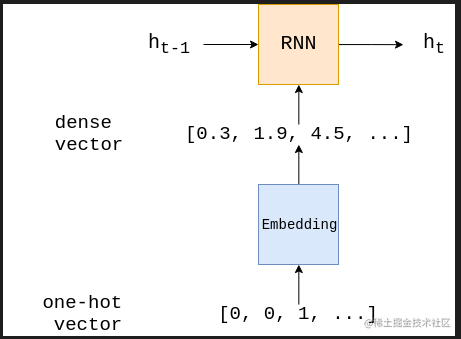```Python import torch.nn as nn

class RNN(nn.Module): def init(self, input_dim, embedding_dim, hidden_dim, output_dim):

``````    super().__init__()

self.embedding = nn.Embedding(input_dim, embedding_dim)

self.rnn = nn.RNN(embedding_dim, hidden_dim)

self.fc = nn.Linear(hidden_dim, output_dim)

def forward(self, text):

#text = [sent len, batch size]

embedded = self.embedding(text)

#embedded = [sent len, batch size, emb dim]

output, hidden = self.rnn(embedded)

#output = [sent len, batch size, hid dim]
#hidden = [1, batch size, hid dim]

assert torch.equal(output[-1,:,:], hidden.squeeze(0))

return self.fc(hidden.squeeze(0))
``````

``` 下面，我们可以做建立一个RNN的例子

embedding 维度是可以设置的超参数. 通常设置为 50-250 维度, 某种程度上也和词典大小有关.

```Python INPUT_DIM = len(TEXT.vocab) #词典大小 EMBEDDING_DIM = 100 HIDDEN_DIM = 256 OUTPUT_DIM = 1

model = RNN(INPUT_DIM, EMBEDDING_DIM, HIDDEN_DIM, OUTPUT_DIM) ``` 也可以输出要训练的参数数目看看.

```Python def count_parameters(model): return sum(p.numel() for p in model.parameters() if p.requires_grad)

print(f'The model has {count_parameters(model):,} trainable parameters') ```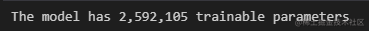## 1.4 训练模型

```Python import torch.optim as optim

optimizer = optim.SGD(model.parameters(), lr=1e-3) ``` 接下来定义损失函数，BCEWithLogitsLoss一般用来做二分类。

```Python criterion = nn.BCEWithLogitsLoss()````.to`, 可以将张量放到gpu上计算。

```Python model = model.to(device) criterion = criterion.to(device)``` 损失函数用来计算损失值，还需要计算准确率的函数。

```Python def binary_accuracy(preds, y): """ Returns accuracy per batch, i.e. if you get 8/10 right, this returns 0.8, NOT 8 """

``````#round predictions to the closest integer
rounded_preds = torch.round(torch.sigmoid(preds)) #四舍五入
correct = (rounded_preds == y).float() #convert into float for division
acc = correct.sum() / len(correct)
return acc
``````

````train` 函数迭代所有的样本，每次都是一个batch。

`model.train()` 将model处于 "training 模式", 也会打开 dropoutbatch normalization. 在每一次的batch, 先将梯度清0. 模型的每一个参数都有一个 `grad` 属性， 存储着损失函数计算的梯度值. PyTorch 不会自动删除（或“归零”）从上次梯度计算中计算出的梯度，因此必须手动将其归零。

`loss.backward()`计算梯度，更新参数使用的是 `optimizer.step()`

```Python def train(model, iterator, optimizer, criterion):

``````epoch_loss = 0
epoch_acc = 0

model.train()

for batch in iterator:

predictions = model(batch.text).squeeze(1) #得到预测

loss = criterion(predictions, batch.label) #计算Loss

acc = binary_accuracy(predictions, batch.label) #计算准确率

loss.backward() #反向传播计算梯度

optimizer.step() #更新参数

epoch_loss += loss.item()
epoch_acc += acc.item()

return epoch_loss / len(iterator), epoch_acc / len(iterator)  #返回整个epoch中损失和准确率的平均值
``````

````evaluate`和`train`相似, 只要将train函数稍微进行修改即可。

`model.eval()` 将模型置于"evaluation 模式", 这会关掉 dropoutbatch normalization.

`with no_grad()` 下，不会进行梯度计算. 这会导致使用更少的内存并加快计算速度.

```Python def evaluate(model, iterator, criterion):

``````epoch_loss = 0
epoch_acc = 0

model.eval()

for batch in iterator:

predictions = model(batch.text).squeeze(1)

loss = criterion(predictions, batch.label)

acc = binary_accuracy(predictions, batch.label)

epoch_loss += loss.item()
epoch_acc += acc.item()

return epoch_loss / len(iterator), epoch_acc / len(iterator)
``````

``` 接下来创建计算每一个epoch会消耗多少时间的函数。

```Python import time

def epoch_time(start_time, end_time): elapsed_time = end_time - start_time elapsed_mins = int(elapsed_time / 60) elapsed_secs = int(elapsed_time - (elapsed_mins * 60)) return elapsed_mins, elapsed_secs ``` 然后，我们通过多个 epoch 来训练模型，每一个 epoch 是对训练和验证集中所有样本的完整传递。

```Python N_EPOCHS = 5

best_valid_loss = float('inf')

for epoch in range(N_EPOCHS):

``````start_time = time.time()

train_loss, train_acc = train(model, train_iterator, optimizer, criterion)
valid_loss, valid_acc = evaluate(model, valid_iterator, criterion)

end_time = time.time()

epoch_mins, epoch_secs = epoch_time(start_time, end_time)

if valid_loss < best_valid_loss:
best_valid_loss = valid_loss
torch.save(model.state_dict(), 'tut1-model.pt')

print(f'Epoch: {epoch+1:02} | Epoch Time: {epoch_mins}m {epoch_secs}s')
print(f'\tTrain Loss: {train_loss:.3f} | Train Acc: {train_acc*100:.2f}%')
print(f'\t Val. Loss: {valid_loss:.3f} |  Val. Acc: {valid_acc*100:.2f}%')
``````

```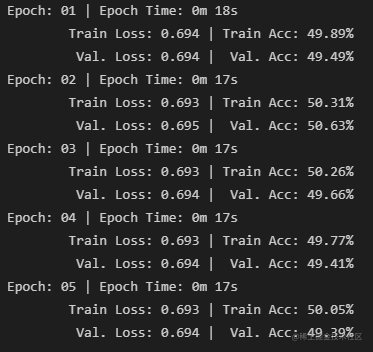如上所示，损失并没有真正减少多少，而且准确性很差。 这是由于这是baseline，我们将在下一个notbook中改进的模型的几个问题。

test_loss, test_acc = evaluate(model, test_iterator, criterion)

print(f'Test Loss: {test_loss:.3f} | Test Acc: {test_acc*100:.2f}%') ```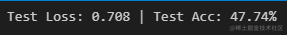「其他文章」